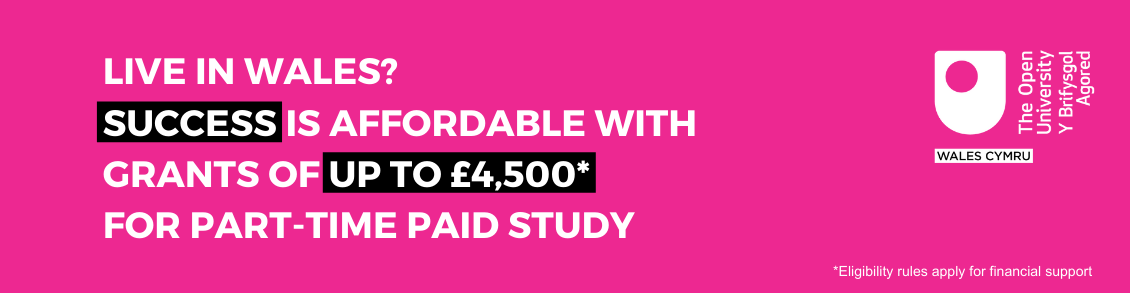Everyday maths 2 (Wales)

Start this free course now. Just create an account and sign in. Enrol and complete the course for a free statement of participation or digital badge if available.

# 3.2 Rounding to approximate an answer

You might round in order to approximate an answer. At the coffee shop, you might want to buy a latte for £2.85, a cappuccino for £1.99 and a tea for £0.99. It is natural to round these amounts up to £3, £2 and £1 in order to arrive at an approximate cost of £6 for all three drinks. It is also very useful when checking calculations to make sure that your answer makes sense, especially when working with large numbers and decimals.

## Activity 9: Approximation

Calculate the following using a calculator and use estimation to check your answers.

1. On 5th March 2019, 190 people matched 5 numbers and won £1650 each. What was the total prize fund?

2. Swansea AFC’s home ground is the Liberty Stadium which holds 21 088 fans. Cardiff City FC plays at the Cardiff City Stadium which holds 33 316 fans.

What is the difference in capacity at these grounds?

1. The actual answer is £313 500 (190 × 1650)

Estimate 200 × 1700.

2 × 17 = 34 so 200 × 1700 = 340 000 so the answer is sensible.

2. The actual answer is 12 228 (33 316 − 21 088)

Estimate 33 000 − 21 000 = 12 000 so the answer is sensible.

## Summary

In this section you have learned:

• how and when to use rounding to approximate an answer to a calculation

• how to round an answer to a given degree of accuracy – e.g. rounding to two decimal places.#### Find out more

*Eligibility rules apply for financial support.